Courses

# Fill Ups of Ray and Wave Optics, Past year Questions JEE Advance, Class 12, Physics JEE Notes | EduRev

## Class 12 Physics 35 Years JEE Mains &Advance Past year Paper

Created by: Abhishek Kapoor

## JEE : Fill Ups of Ray and Wave Optics, Past year Questions JEE Advance, Class 12, Physics JEE Notes | EduRev

The document Fill Ups of Ray and Wave Optics, Past year Questions JEE Advance, Class 12, Physics JEE Notes | EduRev is a part of the JEE Course Class 12 Physics 35 Years JEE Mains &Advance Past year Paper.
All you need of JEE at this link: JEE

Fill in the Blanks

Q.1. A light wave of frequency 5 × 1014 Hz enters a medium of refractive index 1.5. In the medium the velocity of the light wave is ......... and its wavelength is ..........           (1983 - 2 Marks)

Ans. 2 × 108 m/s, 0.4 × 10–6 m

Solution.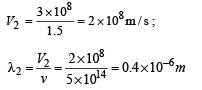Q.2. A convex lens A of focal length 20 cm and a concave lens B of focal length 5 cm are kept along the same axis with a distance d between them. If a parallel beam of light falling on A  leaves B as a parallel beam, then d is equal to ...... cm.            (1985 - 2 Marks)

Ans. d = +15 cm

Solution.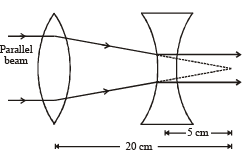From the diagram it is clear that the focus of both the lenses should coincide as shown in the diagram.

Therefore d = 15 cm.

Q.3. A monoch romatic beam of light of wavelength 6000 Å in vacuum enters a medium of refractive index 1.5.  In the medium its wavelength is ....., its frequency is ......          (1985 - 2 Marks)

Ans. 4000Å, 5 × 1014 Hz

Solution. KEY CONCEPT :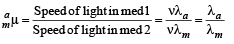[∴ v does not change with the medium]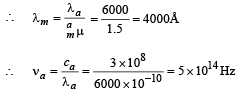Q.4. In Young’s double-slit experiment, the two slits act as coherent sources of equal amplitude ‘A’ and of wavelength ‘λ’. In another experiment with the same set-up the two slits are sources of equal amplitude ‘A’ and wavelength ‘λ’, but are incoherent. The ratio of the intensity of light at the midpoint of the screen in the first case to that in the second case is .............      (1986 - 2 Marks)

Ans. 2

Solution. For coherent sources, for constructive interference The amplitude at the mid point = A + A = 2A

⇒ I1 ∝ (2A)2 ⇒ I2 ∝ 4 I0 ... (i)

NOTE : For incoherent sources, the intensity add up normally (no interference).

Therefore, the total intensity I, = 2I0 ... (ii)

From (i) and (ii)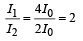Q.5. A thin lens of refractive index 1.5 has a focal length of 15 cm in air. When the lens is placed in a medium of refractive index 4/3,  its focal length will become ........cm.       (1987 - 2 Marks)

Ans. 60 cm

Solution.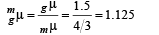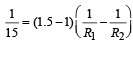[Lensmaker's formula]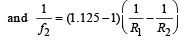On dividing we get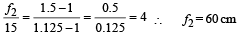Q.6. A point source emits sound equally in all directions in a non-absorbing medium. Two points P and Q are at a distance of 9 meters and 25 meters respectively from the source. The ratio of amplitudes of the  waves at P and Q is ............      (1989 - 2 Marks)

Ans. 25/9

Solution.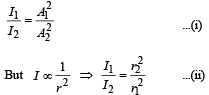From (i) and (ii),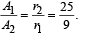Q.7. A slab of a material of refractive index 2 shown in fig. has a curved surface APB of radius of curvature 10 cm and a plane surface CD. On the left of APB is air and on the right of CD is water with refractive indices as given in the figure. An object O is placed at a distance of 15 cm from the pole P as shown. The distance of the final image of O from P, as viewed from the left is ...............      (1991 - 2 Marks)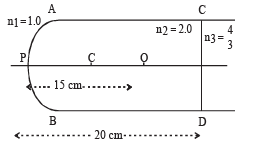Ans. 30 cm

Solution. For refraction at APB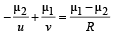⇒ Image of O will be formed at 30 cm to the right at P.

Q.8. A thin rod of length f/3 is placed along the optic axis of a concave mirror of focal length f such that its image which is real and elongated, just touches the rod. The magnification is ....................       (1991 - 1  Mark)

Ans. 1.5

Solution. Since the image formed is real and elongated, the situation is as shown in the figure. Since the image of B is formed at B' itself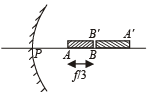∴ B is situated at the centre of curvature that is at a distance at 2f from the pole.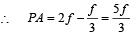Let us find the image of A. For point A,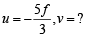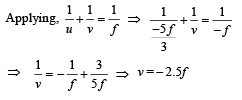Image length = 2.5 f – 2f = 0.5f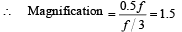Q.9. A ray of light undergoes deviation of 30° when incident on an equilateral prism of refractive index √2. The angle made by the ray inside the prism with the base of the prism is ....................     (1992 - 1 Mark)

Ans. zero

Solution.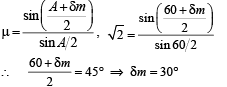The condition is for minium deviation. In this case the ray inside the prism becomes parallel to base. Therefore the angle made by the ray inside the prism with the base of the prism is zero.

Q.10. The resolving power of electron microscope is higher that that of an optical microscope because the wavelength of electrons is ..................... than the wavelength of visible light.          (1992 - 1 Mark)

Ans. smaller

Solution. KEY CONCEPT : The resolving power of  a microscope device is inversely proportional to the wavelength used.

⇒ Ther esolving power of an electr on microscope is higher than that of an optical microscope because the wavelength of electrons is smaller than the wavelength of visible light.

Q.11. If ε0 and μ0 are, respectively, the electric permittivity and magnetic permeability of free space, ε and μ the corresponding quantities in a medium, the index of refraction of the medium in terms of the above parameters is ......................        (1992 - 1 Mark)

Ans.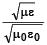Solution. Velocity of light in vacuum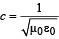and the velocity of light in a medium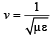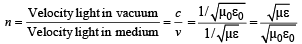Q.12. A light of wavelength 6000Å in air, enters a medium with refractive index 1.5 Inside the medium its frequency is .... Hz and its wavelength is .... Å. (1997 - 2 Marks)

Ans. 5 × 1014 Hz, 4000Å

Solution. Frequency remains the same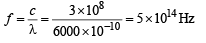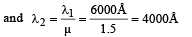Q.13. Two thin lenses, when in contact, produce a combination of power +10 diopters. When they are 0.25 m apart, the power reduces to +6 diopters. The focal length of the lenses are .... m and ... m.       (1997 - 2 Marks)

Ans. 0.125 m, 0.5 m

Solution. P1 + P2 = 10 m– 1

P1 + P2 – (0.25) P1P2 = 6m – 1

From these two expressions, we get

P1 P2 = 16m –2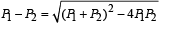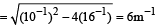∴ P1 = 8m – 1 and P2 = 2 m – 1, Hence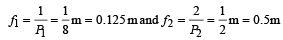Q.14. A ray of light is incident normally on one of the faces of a prism of apex angle 30° and refractive index √2 . The angle of deviation of the ray is... degrees.

Ans. 15°

Solution. Using Snell's law for the refraction at AC, we get µ sin i = (1) sin r

√2 sin 30°= sin r ⇒ r = 45°

Angle of deviation at face AC

= 45° – 30° = 15°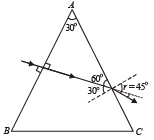Offer running on EduRev: Apply code STAYHOME200 to get INR 200 off on our premium plan EduRev Infinity!

42 docs|19 tests

,

,

,

,

,

,

,

,

,

,

,

,

,

,

,

,

,

,

,

,

,

,

,

,

,

,

,

,

,

,

;